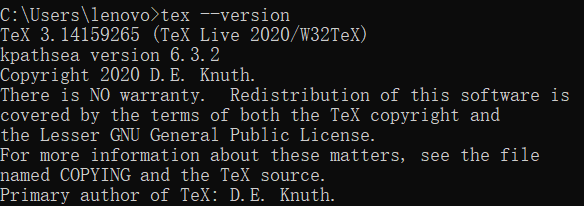y

yellow

V1

2022/07/22阅读：31主题：橙心

# LaTeX编写中文实验进展汇报

## 1 LaTeX学习内容

• 分章节编写中文实验进展
• 包括编写与引用数学符号、公式
• 包括插入与引用图片
• 包括绘制与引用带有注释的三线表格
• 代码下载链接 https://pan.baidu.com/s/1vd13mVXzss3RFSdIw23nFA 提取码：1111
• LaTeX版本与Texstudio版本## 3 LaTeX代码部分

%%%%%%%%%%%%%%%%%%%%%%%%%%%%%%%%%%%%%%%%%%%%%%%%%%%%%%%%%%%%%%%%%%%%%%%%%%%%  1.导入latex文档类与编辑包  %%%%%%%%%%%%%%%%%%%%%%%%%%%%%%%%%%%%%%%%%%%%%%%%%%%%%%%%%%%%%%%%%%%%%%%%%%%%\documentclass[fontset=windows]{article}\usepackage[margin=1in]{geometry}          % 页面布局设置（上下左右边距）\usepackage{ctex}                          % 中文宏包，设置字体族、系列、形状\usepackage{amsmath}                       % 数学公式宏包\usepackage{amssymb}                       % 数学符号宏包\usepackage{amsthm}                        % 定理、定义、证明、例题相关的设置\usepackage{indentfirst}                   % 首行缩进宏包\usepackage{multirow}                      % 表格合并行单元格\usepackage{booktabs}                      % 三线表宏包（3行横线表格）\usepackage{threeparttable}                % 表格添加注释\usepackage{hyperref}                                    % 自动生成目录\hypersetup{colorlinks=true, linkcolor=black}            % 目录设置无红色框线\usepackage{setspace}                                    % 行间距宏包\usepackage{lipsum}                                      % 生成文本随机宏包，没有啥内容写的时候使用\lipsum生成文本\usepackage{graphicx}                                    % 插入图片\usepackage{listings}                                    % 排代码用的宏包\lstset{ columns=fixed,        numbers=left,                                        % 在左侧显示行号 frame=none, %tb,                                     % 不显示背景边框 backgroundcolor=\color[RGB]{244,244,244},            % 设定背景颜色：浅晦涩，近透明 keywordstyle=\color[RGB]{40,40,255},                 % 设定关键字颜色 numberstyle=\footnotesize\color{darkgray},           % 设定行号格式 commentstyle=\it\color[RGB]{0,96,96},                % 设置代码注释的格式 stringstyle=\rmfamily\slshape\color[RGB]{128,0,0},   % 设置字符串格式 showstringspaces=false,                              % 不显示字符串中的空格 language=Python,                                     % 设置语言C, C++, Python... basicstyle=\small, breaklines=true}\newcommand{\refformula}{Equation (\ref{#1})}         % 数学公式引用\newcommand{\reffig}{Figure \ref{#1}}                 % 图片引用\newcommand{\reftable}{Table \ref{#1}}                % 表格引用\newcommand{\refsection}{Section \ref{#1}}            % 主章节引用\newcommand{\refsubsection}{Section \ref{#1}}         % 子章节引用
%%%%%%%%%%%%%%%%%%%%%%%%%%%%%%%%%%%%%%%%%%%%%%%%%%%%%%%%%%%%%%%%%%%%%%%%%%%%%%%%%%%  2.全局字体格式  %%%%%%%%%%%%%%%%%%%%%%%%%%%%%%%%%%%%%%%%%%%%%%%%%%%%%%%%%%%%%%%%%%%%%%%%%%%%%%%%\begin{document}\begin{CJK*}{GBK}{song}              % 宋体为中文字体
%%%%%%%%%%%%%%%%%%%%%%%%%%%%%%%%%%%%%%%%%%%%%%%%%%%%%%%%%%%%%%%%%%%%%%%%%%%%%%%%%%%  3.标题部分  %%%%%%%%%%%%%%%%%%%%%%%%%%%%%%%%%%%%%%%%%%%%%%%%%%%%%%%%%%%%%%%%%%%%%%%%%%%%%%%%%%%%\title{实验进展汇总分析}         % 标题\author{\songti 梁俊超}         % 作者\date{\today}                   % 格式化日期\maketitle                      % 加入此命令后可显示标题\thispagestyle{empty}           % 标题页不加页码
%%%%%%%%%%%%%%%%%%%%%%%%%%%%%%%%%%%%%%%%%%%%%%%%%%%%%%%%%%%%%%%%%%%%%%%%%%%%%%%%%%%  4.目录部分  %%%%%%%%%%%%%%%%%%%%%%%%%%%%%%%%%%%%%%%%%%%%%%%%%%%%%%%%%%%%%%%%%%%%%%%%%%%%%%%%%%%\newpage                        % 目录在新的一页开始\begin{center} \tableofcontents            % 目录居中\end{center}\pagenumbering{Roman}           % 目录设置罗马数字页码\newpage                        % 目录独占一页后，正文开始新的一页
%%%%%%%%%%%%%%%%%%%%%%%%%%%%%%%%%%%%%%%%%%%%%%%%%%%%%%%%%%%%%%%%%%%%%%%%%%%%%%%%%%%  5.章节正文部分  %%%%%%%%%%%%%%%%%%%%%%%%%%%%%%%%%%%%%%%%%%%%%%%%%%%%%%%%%%%%%%%%%%%%%%%%%%%%%%%%\setcounter{page}{1}            % 正文开始页码计数\pagenumbering{arabic}          % 设置阿拉伯数字页码
%%%%%%%%%%%%%%%%%%%%%%%%%%%%%%%%%%%%%%%%%%%%%%%%%%%%%%%%%%%%%%%%%%%%%%%%%%%%%%%%%%%  第1章  %%%%%%%%%%%%%%%%%%%%%%%%%%%%%%%%%%%%%%%%%%%%%%%%%%%%%%%%%%%%%%%%%%%%%%%%%%%%%%%%%%%%%%%%\section{数据集介绍}            % 第1个主章节名称数学符号可以正常使用！集合$A=\{x_i,y_i\},i=1,2,...,n$。
%%%%%%%%%%%%%%%%%%%%%%%%%%%%%%%%%%%%%%%%%%%%%%%%%%%%%%%%%%%%%%%%%%%%%%%%%%%%%%%%%%%  第2章  %%%%%%%%%%%%%%%%%%%%%%%%%%%%%%%%%%%%%%%%%%%%%%%%%%%%%%%%%%%%%%%%%%%%%%%%%%%%%%%%%%%%%%%%\section{算法介绍}              % 第2个主章节名称\subsection{分位数回归}         % 子章节2.1名称中文与英文可编辑！\subsection{模拟推导}           % 子章节2.2名称公式可以生成与引用！\begin{equation}                % 使用equation编辑公式、带编号 a_{1} = \underset{a_1}{arg} \ max \ var(F_{1})=  \underset{a_1}{arg} \ max \ a_1^T\Sigma a_1, \label{PCA3}\end{equation}                  % 使用equation编辑公式、带编号，引用时名称为PCA3 where $a_{1}^{T}a_{1}=1$. According to \refformula{PCA3}, we can use the Lagrange multiplier method to calculate $a_{1}$, by optimizing\begin{eqnarray*}               % 使用eqnarray*编辑公式、无编号 l(a_{1})=a_{1}^{T}var(X)a_{1}- \lambda_1 (a_{1}^{T}a_{1}-1),\end{eqnarray*}                 % 使用eqnarray*编辑公式、无编号where $\lambda_1$ is called the Lagrange factor.
%%%%%%%%%%%%%%%%%%%%%%%%%%%%%%%%%%%%%%%%%%%%%%%%%%%%%%%%%%%%%%%%%%%%%%%%%%%%%%%%%%%  第3章  %%%%%%%%%%%%%%%%%%%%%%%%%%%%%%%%%%%%%%%%%%%%%%%%%%%%%%%%%%%%%%%%%%%%%%%%%%%%%%%%%%%%%%%%\section{实验结果}              % 第3个主章节名称\subsection{图片汇总}           % 子章节3.1名称图片可显示与引用！\begin{figure*}[htb]            % *、htb图片排版尽量好，其他格式自行查找 \includegraphics[width=5in, height=2.4in]{tree}        % tree为保存的图片名称，读取图片文件 \centering \caption{The $m^{th}$ regression decision tree of GBDT  fitting $T_{eff}$ with photometric data.} % caption为图注释内容 \label{tree}                % tree为图片名称，下述引用时直接引用 \centering                  % 图片居中显示\end{figure*}图片如\reffig{tree}所示!figure*图片排版为最佳位置！好看！ \subsection{表格汇总}      % 子章节3.2名称表格可显示与引用！\begin{table*}[htb]\normalsize                  % *、htb表格排版尽量好，其他格式自行查找，normalsize为表格中字体大小 \centering                                  % 字居中 \caption{The model configurations of RF、  and XGBoost using Bayesian  optimization.\label{Tab1}}              % 表名称，用于后面引用 \renewcommand\tabcolsep{15pt}               % 表大小间隔设置 \begin{threeparttable}  \begin{tabular}{*5{c}}                  % 设置5列，内容设置比葫芦画瓢吧，不好解释   \toprule   \multirow{2}{*}{model configurations} & \multicolumn{2}{c}{RF} & \multicolumn{2}{c}{XGBoost} \\   \cmidrule(r){2-3} \cmidrule(r){4-5}   &  set\tnote{1}     & best\tnote{2}   & set\tnote{1}     & best\tnote{2}  \\   \midrule   n\_estimators   &(1,2000)&[1918,948,1610]    &(1,2000)&[1936,1040,1935]    \\   max\_depth  &(1,20)&[18,20,20] &(1,10)&[6,9,8] \\   num\_leaves &(10,5000)&[4014,2169,1835] &$\times$ &$\times$   \\   min\_child\_samples &(1,20)&[3,1,4] &$\times$&$\times$  \\   min\_samples\_split   &(2,20)&[4,2,2] &$\times$&$\times$  \\   learning\_rate   &$\times$&$\times$ &(0.001,0.3)&[0.037,0.023,0.017]  \\   subsample     &$\times$&$\times$  &(0.1,1)&[0.46,0.27,0.34]   \\   colsample\_bytree  &$\times$&$\times$  &(0.1,1)&[0.81,0.99,0.94] \\   colsample\_bynode  &$\times$&$\times$  &(0.1,1)&[0.95,0.51,0.41] \\   reg\_lambda  &$\times$&$\times$  &(0,1)&[1,0.15,0.34] \\   \bottomrule  \end{tabular}  \begin{tablenotes}    % 添加表内容注释   \footnotesize   \item Set indicates the value range of the   hyper-parameters, $\times$ means that the model does not have this hyper-parameter.   \item Best represents the best hyper-parameters of the model for $T_{eff}$, $\log \ g$ and [Fe/H] respectively, $\times$ means that the model does not have this hyper-parameter.  \end{tablenotes}      % 添加表内容注释 \end{threeparttable}\end{table*} 表格如\reftable{Tab1}所示！table*表格排版为最佳位置，好看！\subsection{实验结论}      % 子章节3.3名称实验结果可列举！如下两条！ \begin{enumerate} \item Estimating stellar atmospheric parameters directly by the OPD or CID is difficult, because the strong MIC between variables leads to the instability of the model and inability to correctly identify the important features of stellar atmospheric parameters. \item  PCA can eliminate the MIC between new variables through weighted sum of variables and enhance the MIC between new variables and stellar atmospheric parameters, making the final model more stable and the estimation more accurate.\end{enumerate} 
%%%%%%%%%%%%%%%%%%%%%%%%%%%%%%%%%%%%%%%%%%%%%%%%%%%%%%%%%%%%%%%%%%%%%%%%%%%%%%%%%%%  第4章  %%%%%%%%%%%%%%%%%%%%%%%%%%%%%%%%%%%%%%%%%%%%%%%%%%%%%%%%%%%%%%%%%%%%%%%%%%%%%%%%%%%%%%%% \section{问题分析}              % 第4个主章节名称自己的问题列举出来，提问老师们，下次汇报使用重点！\begin{itemize} \item Estimating stellar atmospheric parameters directly by the OPD or CID is difficult, because the strong MIC between variables leads to the instability of the model and inability to correctly identify the important features of stellar atmospheric parameters. \item  PCA can eliminate the MIC between new variables through weighted sum of variables and enhance the MIC between new variables and stellar atmospheric parameters, making the final model more stable and the estimation more accurate.\end{itemize} 
\end{CJK*}\end{document}

y
V1